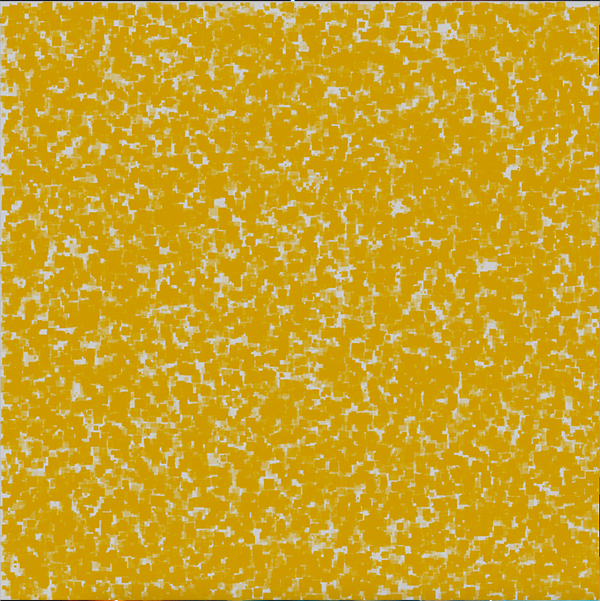# PImage, and color in Processing

I wanted to explore little bit with the PImage object in Processing. Below, are some variations of studies using PImage object.

`//Load an image and then display it`
```PImage img; void setup(){ size(950, 950,P3D); img = loadImage("yourimage.jpg"); }```

```void draw(){ image(img, 0, 0); }```Now. let’s think of a simple animation. Maybe, we’ll start with some random squares in the screen, we’ll give them a color, and we’ll define some opacity.

```void setup(){ size(950, 950,P3D); }```
```void draw(){ float x = random(width); float y = random(height); float size = random(1,16); color c = color(204, 153, 0); fill(c, random(255)); noStroke(); rect(x,y,size,size); }```

Also, you can put a loop in the drawing function. The rectangles will populate faster the stage.

``` for (int i =0; i<20; i++){ float x = random(width); float y = random(height); float size = random(1,16); color c = color(204, 153, 0); fill(c, random(255)); noStroke(); rect(x,y,size,size); }```Why not render the image color information into the ractangles. See code below

```PImage img; void setup(){ size(950, 950,P3D); img = loadImage("img.png"); background(0); } void draw(){ for (int i =0; i<100; i++){ float x = random(width); float y = random(height); float size = random(1,16); color c = img.get(int(x),int(y));//store the color from the image fill(c, random(255));//color the rectangles with the image colors noStroke(); rect(x,y,size,size); } if (frameCount % 700 == 0) { background(255);//reset the stage } }```